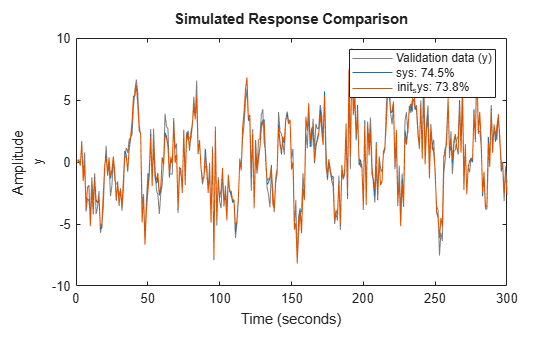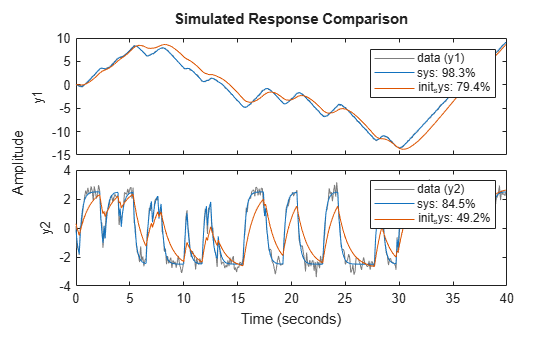Documentation

pem

Prediction error estimate for linear and nonlinear model

Description

example

sys = pem(data,init_sys) updates the parameters of an initial model to fit the estimation data. The function uses prediction-error minimization algorithm to update the parameters of the initial model. Use this command to refine the parameters of a previously estimated model.

example

sys = pem(data,init_sys,opt) specifies estimation options using an option set.

Examples

collapse all

Estimate a discrete-time state-space model using the subspace method. Then, refine it by minimizing the prediction error.

Estimate a discrete-time state-space model using n4sid, which applies the subspace method.

z7a = z7(1:300);
opt = n4sidOptions('Focus','simulation');
init_sys = n4sid(z7a,4,opt);

init_sys provides a 73.85% fit to the estimation data.

init_sys.Report.Fit.FitPercent
ans = 73.8490

Use pem to improve the closeness of the fit.

sys = pem(z7a,init_sys);

Analyze the results.

compare(z7a,sys,init_sys);sys provides a 74.54% fit to the estimation data.

Estimate the parameters of a nonlinear grey-box model to fit DC motor data.

Load the experimental data, and specify the signal attributes such as start time and units.

data = iddata(y, u, 0.1);
data.Tstart = 0;
data.TimeUnit = 's';

Configure the nonlinear grey-box model (idnlgrey) model.

For this example, use dcmotor_m.m file. To view this file, type edit dcmotor_m.m at the MATLAB® command prompt.

file_name = 'dcmotor_m';
order = [2 1 2];
parameters = [1;0.28];
initial_states = [0;0];
Ts = 0;
init_sys = idnlgrey(file_name,order,parameters,initial_states,Ts);
init_sys.TimeUnit = 's';

setinit(init_sys,'Fixed',{false false});

init_sys is a nonlinear grey-box model with its structure described by dcmotor_m.m. The model has one input, two outputs and two states, as specified by order.

setinit(init_sys,'Fixed',{false false}) specifies that the initial states of init_sys are free estimation parameters.

Estimate the model parameters and initial states.

sys = pem(data,init_sys);

sys is an idnlgrey model, which encapsulates the estimated parameters and their covariance.

Analyze the estimation result.

compare(data,sys,init_sys);sys provides a 98.34% fit to the estimation data.

Create a process model structure and update its parameter values to minimize prediction error.

Initialize the coefficients of a process model.

init_sys = idproc('P2UDZ');
init_sys.Kp = 10;
init_sys.Tw = 0.4;
init_sys.Zeta = 0.5;
init_sys.Td = 0.1;
init_sys.Tz = 0.01;

The Kp, Tw, Zeta, Td, and Tz coefficients of init_sys are configured with their initial guesses.

Use init_sys to configure the estimation of a prediction error minimizing model using measured data. Because init_sys is an idproc model, use procestOptions to create the option set.

opt = procestOptions('Display','on','SearchMethod','lm');
sys = pem(z1,init_sys,opt);

Examine the model fit.

sys.Report.Fit.FitPercent
ans = 70.6330

sys provides a 70.63% fit to the measured data.

Input Arguments

collapse all

Estimation data that contains measured input-output data, specified as an iddata or idfrd object. You can use frequency-domain data only when init_sys is a linear model.

The input-output dimensions of data and init_sys must match.

Identified model that configures the initial parameterization of sys, specified as a linear, or nonlinear model. You can obtain init_sys by performing an estimation using measured data or by direct construction.

init_sys must have finite parameter values. You can configure initial guesses, specify minimum/maximum bounds, and fix or free for estimating any parameter of init_sys:

• For linear models, use the Structure property. For more information, see Imposing Constraints on Model Parameter Values.

• For nonlinear grey-box models, use the InitialStates and Parameters properties. Parameter constraints cannot be specified for nonlinear ARX and Hammerstein-Wiener models.

Estimation options that configure the algorithm settings, handling of estimation focus, initial conditions, and data offsets, specified as an option set. The command used to create the option set depends on the initial model type:

Output Arguments

collapse all

Identified model, returned as the same model type as init_sys. The model is obtained by estimating the free parameters of init_sys using the prediction error minimization algorithm.

Algorithms

PEM uses numerical optimization to minimize the cost function, a weighted norm of the prediction error, defined as follows for scalar outputs:

${V}_{N}\left(G,H\right)=\sum _{t=1}^{N}{e}^{2}\left(t\right)$

where e(t) is the difference between the measured output and the predicted output of the model. For a linear model, the error is defined as:

$e\left(t\right)={H}^{-1}\left(q\right)\left[y\left(t\right)-G\left(q\right)u\left(t\right)\right]$

where e(t) is a vector and the cost function ${V}_{N}\left(G,H\right)$ is a scalar value. The subscript N indicates that the cost function is a function of the number of data samples and becomes more accurate for larger values of N. For multiple-output models, the previous equation is more complex. For more information, see chapter 7 in System Identification: Theory for the User, Second Edition, by Lennart Ljung, Prentice Hall PTR, 1999.

Alternative Functionality

You can achieve the same results as pem by using dedicated estimation commands for the various model structures. For example, use ssest(data,init_sys) for estimating state-space models.